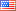# Revision history [back]

### How to return only the denominators of a sequence of fractions created by a formula

With essential help from slelievre, I managed to achieve coding to return the fractional 'density' (if that is the right term) of primes between n^2 and (n+1)^2:

for n in range(1, 51):

a = n^2 + 1

b = (n + 1)^2 + 1

c = 2*n

nprimes = sum(1 for p in prime_range(a, b))

d = f"{nprimes}/{c}"

print(d)


Now I want to extract just the denominators of this sequence so that I can find the LCM of all those denominators and then convert all the fractions to have the same LCM denominator. (I cannot just use 2n to find the LCM because the fractions need to be reduced.) I found the command x.denominator(), but it seems to work for only a single fraction of integers. At least that's what I understand the error message to mean when I try d.denominator() or print(d.denominator()), the error message reading "AttributeError: 'str' object has no attribute 'denominator'".

Any help in showing me how to do this would be greatly appreciated.2 NoneJohn Palmieri6582 ●18 ●57 ●146 http://www.math.washin...

### How to return only the denominators of a sequence of fractions created by a formula

With essential help from slelievre, I managed to achieve coding to return the fractional 'density' (if that is the right term) of primes between n^2 and (n+1)^2:

for n in range(1, 51):
a = n^2 + 1
b = (n + 1)^2 + 1
c = 2*n
nprimes = sum(1 for p in prime_range(a, b))
d = f"{nprimes}/{c}"
print(d)


Now I want to extract just the denominators of this sequence so that I can find the LCM of all those denominators and then convert all the fractions to have the same LCM denominator. (I cannot just use 2n to find the LCM because the fractions need to be reduced.) I found the command x.denominator(), but it seems to work for only a single fraction of integers. At least that's what I understand the error message to mean when I try d.denominator() or print(d.denominator()), the error message reading "AttributeError: 'str' object has no attribute 'denominator'".

Any help in showing me how to do this would be greatly appreciated.